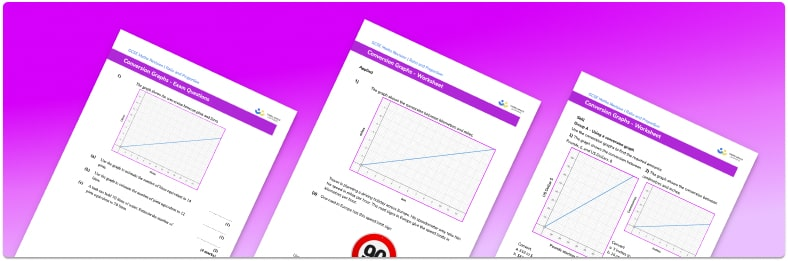# Conversion Graphs Worksheet• Section 1 of the conversion graphs worksheet  worksheet contains 12 skills-based conversion graphs questions, in 3 groups to support differentiation
• Section 2 contains 4 applied conversion graphs questions with a mix of word problems and deeper problem solving questions
• Section 3 contains 4 foundation and higher level GCSE exam questions including word problems
• Answers and a mark scheme for all exam questions are provided
• Questions follow variation theory with plenty of opportunities for students to work independently at their own level
• All questions are created by fully qualified expert secondary maths teachers
• Suitable for GCSE maths revision for AQA, OCR and Edexcel exam boards

• This field is for validation purposes and should be left unchanged.

You can unsubscribe at any time (each email we send will contain an easy way to unsubscribe). To find out more about how we use your data, see our privacy policy.

### Conversion graphs at a glance

Conversion graphs are graphs which are plotted to allow us to easily convert between two units of measurement. For example, a conversion graph could be plotted for converting the imperial units of inches to the metric units of centimetres

The conversion factor between two units of measurement is represented by the gradient of a conversion graph. To plot a conversion graph, we can use the conversion factor to calculate two or three conversions between the two units and then plot these onto the graph before joining them with a straight line

We can use a conversion graph to make conversions. Let’s say we have a conversion graph showing US dollars along the horizontal axis and UK pounds along the vertical axis. To convert a given number of dollars to pounds, we would draw a vertical line from that value on the horizontal axis to the conversion line. We would then draw a horizontal line from that point to the vertical axis and read off the value in pounds.

Looking forward, students can then progress to additional exchange rates and conversion rates worksheet and other ratio and proportion worksheets, for example a ratio worksheet or a simplifying and equivalent ratios worksheetFor more teaching and learning support on Ratio and Proportion our GCSE maths lessons provide step by step support for all GCSE maths concepts.

When you have students who require more intensive support our one to one GCSE maths revision programme will match them with the most appropriate tutor. This way we can provide individual students with personalised programmes of study while you continue to teach the rest of your class as a whole group.

Our maths interventions are currently only available for GCSE students, and are not suitable for A level students.

## Do you have KS4 students who need more focused attention to succeed at GCSE?There will be students in your class who require individual attention to help them succeed in their maths GCSEs. In a class of 30, it’s not always easy to provide.

Help your students feel confident with exam-style questions and the strategies they’ll need to answer them correctly with our dedicated GCSE maths revision programme.

Lessons are selected to provide support where each student needs it most, and specially-trained GCSE maths tutors adapt the pitch and pace of each lesson. This ensures a personalised revision programme that raises grades and boosts confidence.

Find out more